Next: Future Work Up: Interpolation Previous: Quaternion interpolation

BSpline interpolation

one dimensional spline example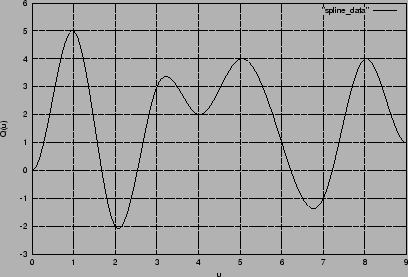The BSpline interpolation is implemented as described in [watt92]. Input is a set of n one dimensional data points Xi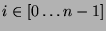and a knot vector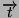containing n+6 knots. A set of n+2 control points pi are generated so that a cubic spline with those control points will fit the data. The control points are such that p0=p1 and pn+1=pn+2. We also subject the curve to the constraint that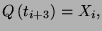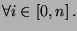This means that the curve is defined over the interval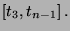This system of equations is then solved to get the control points for the curve.

The curve at time u is given by a weighted sum of the control points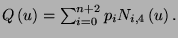The Blending functions are as follows: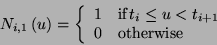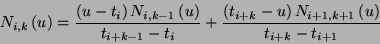Higher dimensional interpolants can be generated by interpolating each element independently.

In general, the interpolation of a parameter proceeds as follows:

control_points = get_control_points(data, knots);

interpolant = spline_at(t, control_points);

brian martin
1999-06-23